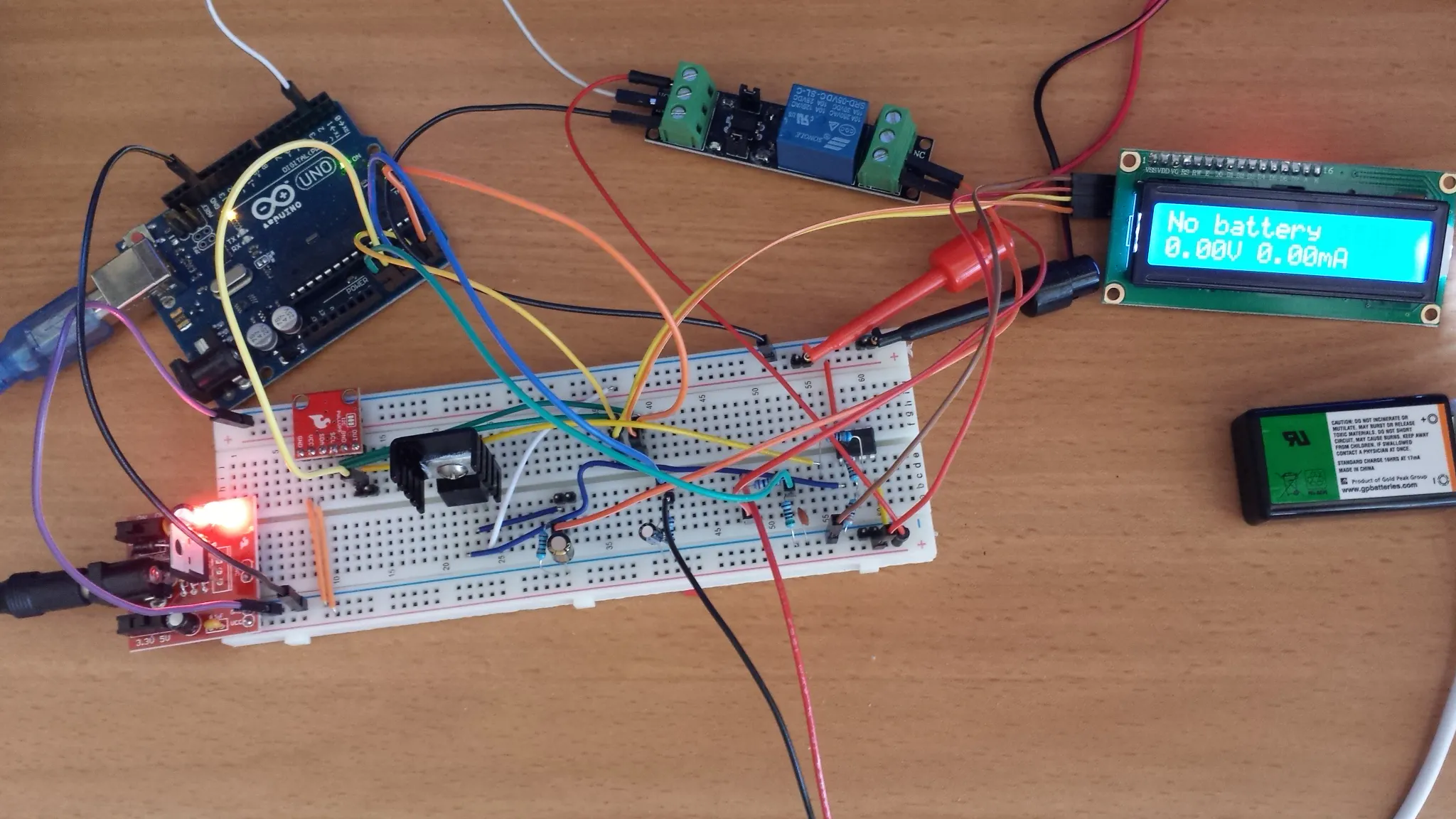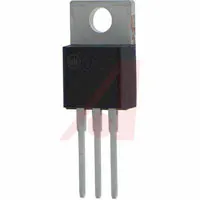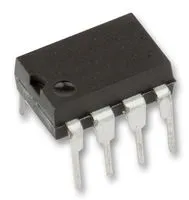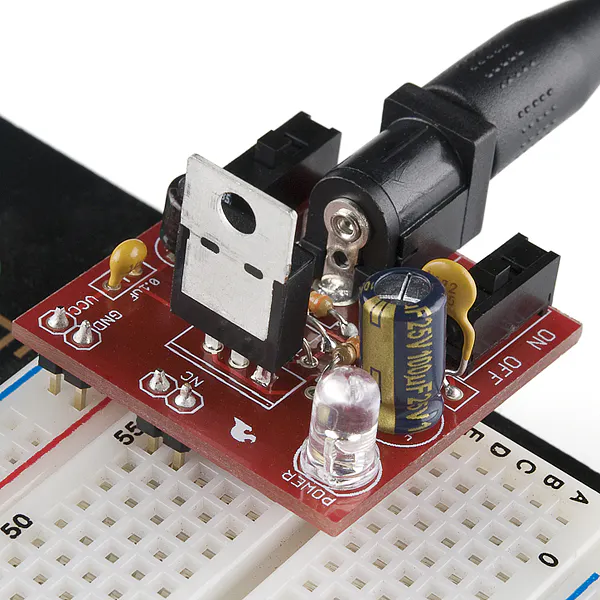Project tutorial# Intelligent Charger for 9V NiMH Rechargeable Batteries V1 © GPL3+

Regulated smart constant current charger for 9V NiMH rechargeable batteries.

• 13,620 views
• 25 respects

## Components and suppliesArduino UNO & Genuino UNO
×1
 SparkFun I2C DAC Breakout - MCP4725
×1Linear Regulator with Adjustable Output
×1Texas Instruments General Purpose Dual Op-Amp
×1
 Resistor 6.8k ohm
×2
 Resistor 3.3k ohm
×1
 Resistor 5.1k ohm
×1Resistor 1k ohm
×1
 Resistor 10 ohm
×1
 Relay (generic)
×1
 Resistor 20k ohm
×1SparkFun Breadboard Power Supply 5V/3.3V
×1
 Power supply 17V
×1
 YwRobot I2C SERIAL LCD 1602 MODULE
×1

I looked for smart charger chat can charge 9V NiMH battery in couple of hours and didn't found one. Moreover all chargers I found was really "dumb". Charging current unknown and no function to terminate charging after battery fully charged. Problem with such chargers that they can overcharge battery and significantly reduce lifespan. So I decided to create "smart" charger.

First version I intend to keep simple, so it allows basic things like charging with constant current, automatic charging termination after battery fully charged, trickle charge, measurement of charge transferred to battery.

In next version I will add couple of additional useful features like discharge, capacity measurement and cycling.

Warning: Charging battery with high current may cause battery explosion or fire. Please don't leave charger unattended. Also please don't try to charge battery don't intended to be charged as Alkaline. This charger tested only with NiMH batteries (and still you are using it at your own risk and I am absolutely have no any responsibility if any damage caused because of bugs in design or code). Chagrin of other types of batteries will require code modification.

### Theory

Some useful facts to remember that will help to understand needed parameters of charger.

C - current equal to nominal capacity of battery

When charged at the C rate single cell voltage may reach 1.6V. This voltage may be higher for old batteries.

Nominal voltage of single cell is 1.2V, but fully charged cell has an open-circuit voltage up to 1.5 volt.

Trickle charge rate of less than 0.025 C (C/40) is recommended after battery fully charged.

It is generally two options to charge NiMH battery:

1. Fast charging. Charging current 0.5C-1C. Charge state must be monitored and terminated by dV/dt (voltage change rate) or dT/dt (temperature change rate)

2. Slow charging. Charging current 0.1C. Charging time 14-16 hours. Charge termination by timer. dT/dt charge termination impossible for low currents. dV/dt termination may be not reliable for currents below 0.5C according to literature.

### Basic Parameters of Charging Circuit

9V battery usually has 7 serially connected sells, but in some cases it may have 6 or 8 cell. Voltage regulator should be able to provide charging voltage at least up to 8*1.6=12.8V. Dropout voltage of LM317 regulator up to 2V, so supply voltage need to be ~15V (this not taking to account voltage drop on current sensing resistor).

For maximum charging current of 200mA and current sensing resistor 10 Ohm additional drop on current sensing resistor is 2V, so 17V supply voltage needed.

Fully discharged cell may have very low, even negative voltage. Minimum voltage of regulator ideally should be 0, but using LM317 it may be as low as 1.2V.

### Circuit Explanations

The basic idea is to measure charging current and adjust voltage of regulator until desired current reached. Current measured by measuring voltage drop on current sensing resistor R5. I=V/R.

SparkFun I2C DAC Breakout - MCP4725 - 12 bit digital to analog converter used to control voltage. Output voltage cam be configured via I2C between 0 and 5V. Because we need to be able to adjust voltage in wider range, from 0 to 15V operational amplifier LM358 used to amplify output voltage of DAC. Amplification of operational amplifier set by resistors R4 and R3. Gain=1+R4/R3=1+6800/3300=3.06 so output voltage of operational amplifier approximately 0 to 15V.

Maximum output current of LM358 is 50mA, so LM317 adjustable voltage regulator used to control higher current. Output of operational amplifier connected to ADJ terminal of LM317. LM317 will maintain 1.2V between ADJ and OUT terminals, so actual voltage on battery can be configured between 1.2 and 16.2V. LM317 need minimum 3.5mA current to maintain regulation. So 1kOhm resistor R6 used to ensure regulation if battery not connected. Capacitor C1 used to filter output voltage and improve stability of LM317.

Voltage measured at two different points.

1. Resistor R5 connected to pin A2 of Arduino. Voltage on resistor measured and than charging current calculated as Icharging=V/R

2. Voltage on battery can be up to 16.2V, so resistive divider R1,R2 used to bring voltage below 5V, allowed by Arduino. Output of divider connected to pin A0 of Arduino. For R1=5.1k Ohm and R2=20kOhm Vout=Vin/(20000+5100)*5100=0.2 So battery voltage divided by 5.

Relay used to disconnect battery from charging circuit. You can see on photo relay I used, but generally any relay with 5V control can be used. It is more safe to connected battery to normally open contacts of relay.

I used YwRobot I2C SERIAL LCD 1602 MODULE to display status of charger, but any other I2C controlled LCD module can be used. It seems like YwRobot LCD module not supported by standard LiquidCrystal_I2C library, so I used New LiquidCrystal library If you are using different LCD module you will need to change this line:

``````LiquidCrystal_I2C lcd(0x27, 2, 1, 0, 4, 5, 6, 7, 3, POSITIVE);  // set the LCD address to 0x27 for a 16 chars and 2 line display
``````

To power Digital to analog converter and LCD I used SparkFun Breadboard Power Supply 5V/3.3V. It is probably will be ok to use 5V from Arduino board.

You also will need to supply 17V to charging circuit. If you don't have power supply, you can use adjustable DC/DC converter like this

### Functionality

I don't wanted a lot of wires, so there are no buttons to configure charging. Chagrin current configured only in code. You need to set desired charging current in charger.ino

``````//*************************** Charging parameters *****************************************
//*****************************************************************************************
float target_current_mA=30;				//Charging current mA
float battery_nominal_capacity_mA=170;	//Nominal capacity of battery mA
float max_time_for_trickle_charge=6;  //Maximum trickle charge time in minutes
//*****************************************************************************************
//*****************************************************************************************
``````

target_current_mA - constant charging current

max_time_for_trickle_charge - maximum number of minutes for trickle charging, can be set up to 600 (10h)

battery_nominal_capacity_mA - battery capacity used to calculate trickle current

Generally charging current can be up to nominal capacity. For battery with nominal capacity of 170mAh maximum charging current is 170mA. Minimum charging current usually C/10 - 17mA for 170mAh battery.

After power is up charger will check if battery connected. If battery connected battery will be charged with configured constant current until fully charged. Charging terminated by detecting negative dV/dt during 5 minutes. After charging completed charger will switch to trickle charging with current C/40. Charger will disconnect itself from battery after maximum trickle charging time elapsed.

1 - dV/dt

2 - charging time in minutes

1 - Charging time

2 - Charge transferred to battery

## Code

##### charger.inoArduino
Main file
```//THis for charging 9V NiMH battery
//Battery can have have 6,7 or 8 1.25V cells
//This makes nominal voltage between 7.5 and 10V

#include <Wire.h>
#include <LiquidCrystal_I2C.h>
#include <TimeLib.h>
#include <TimerOne.h>
#include <elapsedMillis.h>

LiquidCrystal_I2C lcd(0x27, 2, 1, 0, 4, 5, 6, 7, 3, POSITIVE);  // set the LCD address to 0x27 for a 16 chars and 2 line display

//Hardware defenitions
#define DAC_REF_VOLTAGE 5.0
#define CHARGE_RELAY_PIN 2
#define DISCONNECT_CHARGE_RELAY HIGH
#define CONNECT_CHARGE_RELAY LOW
#define CURRENT_SENSING_SESISTOR_PIN A2
#define CURRENT_SENSING_SESISTOR 10.2 //OHm
#define BATTERY_VOLTAGE_PIN A0
#define R1 5090 //Low side resistor of voltage sensing divider
#define R2 19910//High side resistor of voltage sensing divider
#define ADC_REF_VOLTAGE 4.89 //Arduino real supply voltage or AREF voltage for proper ADC to voltage translation
#define R3 3300.0
#define R4 6800.0
#define AMP_GAIN (1+R4/R3)

//Various definitions
//#define MINIMUM_VOLTAGE 8.1 //Minimum voltage after regulator
#define BATTERY_GOOD_VOLTAGE_THRESHOLD 7.0
#define MAXIMIM_ALLOWED_CURRENT 200 //Maximum allowed battery current mA (hard cut-off)
#define MAXIMUM_BATTERY_VOLTAGE 15.0 //Maximum allowed battery voltage V (hard cut-off)
#define VOLTAGE_STEP 0.001 //Step for voltage regulation
#define POINTS_FOR_AVERAGING 100 //How many points taken for averaging
#define MEASURE2MEASURE_US 5000 //Time between measurments in microseconds (need be more then 200 becouse of two analog reads)
#define VOLTAGE_LOG_TIME_MIN 30.0 //Time in minutes to save voltage for dV/dT cutoff
#define MINUTES_TO_LOG 5
#define TRICKLE_CURRENT_RATIO 40

//Charger states
#define INITIALIZATION 0
#define NO_BATTERY 5
#define BATTERY_CONNECTED 10
#define BATTERY_VOLTAGE_LOW 15
#define CURRENT_RAMP_UP 20
#define CHARGING 30
#define CURRENT_RAMP_DOWN 32
#define TRICKLE 35
#define END_OF_TRICKLE 37
#define UNEXPECTED_CURRENT_FALL 40
#define UNABLE_TO_REACH_CURRENT 60
#define FULLY_CHARGED 70
#define CURRENT_TOO_HIGH 80
#define REGULATION_FAILED 90
#define OVERCURRENT 100
#define OVERVOLTAGE 101
#define FINAL_STATE 200

///
long unsigned int voltage_sum,current_sum;//Sum of averaging
float regulator_voltage_code,resistor_voltage,csr_voltage_code,regulator_voltage,current_mA,battery_voltage;//Measurments
float tmp_resistor_voltage,tmp_current_mA,tmp_regulator_voltage,tmp_battery_voltage;
int i,j,charger_state;
short unsigned int last_second,lcd_last_second,log_last_second;
String lcd_last_string1,lcd_last_string2;
String empty_string="";
String msg,eoc_line1,eoc_line2;
unsigned char sec_index,min_index;
//long long int charging_started;
float sec_log,min_log[MINUTES_TO_LOG],last_blf;
float trickle_current_mA;
int total_minutes_average=0;

elapsedMillis ChargingTimeMillis,TrickleChargingTimeMillis;

float wanted_dac_voltage=0;
float last_dac_voltage=0;

//Messages
const char msg_battery_detected[] PROGMEM 		="Battery detected";
const char msg_no_battery[] PROGMEM				="No battery";
const char msg_battery_ok[] PROGMEM				="Battery ok";
const char msg_voltage_too_low[] PROGMEM		="Battery voltage too low";
const char msg_voltage_too_low_short[] PROGMEM	="V Battery low";
const char msg_ramp_up[] PROGMEM				="Ramp up";
const char msg_charging[] PROGMEM				="Charging";
const char msg_space[] PROGMEM					=" ";
const char msg_ramp_down[] PROGMEM				="Ramp down";
const char msg_trickle_charge[] PROGMEM			="Trickle charge";
const char msg_no_current[] PROGMEM				="No current";
const char msg_current_unreachable[] PROGMEM	="I unreachable";
const char msg_current_unreachable_long[] PROGMEM	="Unable to reach desired current";
const char msg_completed[] PROGMEM				="Completed";
const char msg_charge[] PROGMEM					="Charge";
const char msg_high_current[] PROGMEM			="High current";
const char msg_regulation_fault[] PROGMEM		="Regulation fault";
const char msg_overcurrent[] PROGMEM			="Current too high";
const char msg_overvoltage[] PROGMEM			="Voltage too high";
const char msg_trickle_completed[] PROGMEM		="Trickle finished";

//*************************** Charging parameters *****************************************
//*****************************************************************************************
float target_current_mA=30;				//Charging current mA
float battery_nominal_capacity_mA=170;	//Nominal capacity of battery mA
float max_time_for_trickle_charge=6;  //Maximum trickle charge time in minutes
//*****************************************************************************************
//*****************************************************************************************

struct mytime {
unsigned char hours;
unsigned char minutes;
unsigned int total_minutes;
} elapsed_time;

void setup() {
pinMode(CHARGE_RELAY_PIN, OUTPUT);
disconnect_charging_circuit();	//Disconnect charger ftom battery
Wire.begin();//I2C
dac_write_voltage(0);//Make sure lower possible current set

Serial.begin(115200);
last_second=second();
lcd_last_second=second();
log_last_second=second();

Timer1.initialize(MEASURE2MEASURE_US); //Will use to measure voltage and current of battery (microseconds)
Timer1.attachInterrupt(read_hw);  // attaches read_hw() as a timer overflow interrupt
averaging_index=0;
sec_index=0;
min_index=0;
charger_state=0;//Initial state of state machine
wanted_dac_voltage=0;//Make shure minimum voltage outout
last_blf=1.0;
trickle_current_mA=battery_nominal_capacity_mA/TRICKLE_CURRENT_RATIO;
//ChargingTimeMillis=0;
//LCD
lcd.begin(16,2);
lcd.backlight();
lcd.clear();
update_lcd(F("Power up..."),empty_string);
delay(1000);
}

float log_battery_voltage(){
//Log only once per second
if (log_last_second==second()) return last_blf;
else log_last_second=second();

sec_log[sec_index]=battery_voltage;
if (sec_index<59) {
sec_index++;
}
else {//If one minute logged
//Calculate avetage per minute
if (min_index>=MINUTES_TO_LOG) min_index=0;

sec_index=0;
float sum_v=0;
for (i=0;i<60;i++){
sum_v+=sec_log[i];
}
float min_average=sum_v/60.0;

for(i=1;i<MINUTES_TO_LOG;i++) min_log[i-1]=min_log[i];

min_log[MINUTES_TO_LOG-1]=min_average;//Save minute average

//Serial.print(min_index);
//Serial.print(" ");
//Serial.print(min_average);
//Serial.print(" arr: ");
//Serial.print(min_log);
//Serial.print(" ");
//Serial.print(min_log);
//Serial.print(" ");
//Serial.print(min_log);
//Serial.print(" ");
//Serial.print(min_log);
//Serial.print(" ");
//Serial.print(min_log);
last_blf=best_linear_fit(min_log);
//Serial.print("Slope ");
//Serial.println(last_blf);
min_index++;
total_minutes_average++;
}
return last_blf;
}
```
##### main.inoArduino
```void loop() {
String msg1;
switch(charger_state){
case INITIALIZATION://Initial state
disconnect_charging_circuit();//Make shure relay disconnected
dac_write_voltage(0);//Make sure lower possible current set
wanted_dac_voltage=0;//Make shure minimum voltage outout
delay(100);
if (battery_voltage>0.1) {
charger_state=BATTERY_CONNECTED;//Battery detected
update_lcd(M2S(msg_battery_detected),empty_string);
Serial.println(M2S(msg_battery_detected));
delay(2000);
}
else {//No battery
Serial.println(M2S(msg_no_battery));
update_lcd(M2S(msg_no_battery),construct_status_string());
charger_state=NO_BATTERY;//Battery detected
delay(1000);
}
break;
case NO_BATTERY://No battery
if (battery_voltage>0.1) {
charger_state=BATTERY_CONNECTED;//Battery detected
Serial.println(M2S(msg_battery_detected));
update_lcd(M2S(msg_battery_detected),construct_status_string());
delay(1500);
}
else{
//If no battery stay in this state
update_lcd(M2S(msg_no_battery),construct_status_string());
delay(1100);
}
break;
case BATTERY_CONNECTED://Battery connected
dac_write_voltage(0);//Make sure lower possible current set
wanted_dac_voltage=0;
delay(100);
if (battery_voltage>BATTERY_GOOD_VOLTAGE_THRESHOLD){
charger_state=CURRENT_RAMP_UP;//Start charging current rampup
//snprintf(welcome, sizeof(welcome),"Firmware:V%d.%d%d",ver,ver2,ver3);
update_lcd(M2S(msg_battery_ok),construct_status_string());
Serial.println(M2S(msg_battery_ok));
delay(2000);
wanted_dac_voltage=get_approximated_dac_voltage(battery_voltage);//Set needed regulator voltage
//Serial.println(get_approximated_dac_voltage(battery_voltage));
connect_charging_circuit();
delay(200);
}
else {
charger_state=BATTERY_VOLTAGE_LOW;//Battery voltage too low
Serial.println(M2S(msg_voltage_too_low));
update_lcd(M2S(msg_voltage_too_low_short),construct_status_string());
delay(1000);
}
break;
case BATTERY_VOLTAGE_LOW://Battery voltage too low
update_lcd(M2S(msg_voltage_too_low_short),construct_status_string());
Serial.println(M2S(msg_voltage_too_low));
charger_state=FINAL_STATE;//Halt
break;
case CURRENT_RAMP_UP:///Current rampup
//if (current_mA<1.0) charger_state=40;//Current unexpectedly fell
update_lcd(M2S(msg_ramp_up),construct_status_string());
delay(50);
if (current_mA<target_current_mA) {
if (wanted_dac_voltage<4.99) {
wanted_dac_voltage=wanted_dac_voltage+VOLTAGE_STEP;
dac_write_voltage(wanted_dac_voltage);
}
else charger_state=UNABLE_TO_REACH_CURRENT;//Unable to reach desired current
}
else {
charger_state=CHARGING;//Charging
ChargingTimeMillis=0;//Reset counter
}
break;
case CHARGING://Charging
delay(200);
if (current_mA<1.0) charger_state=UNEXPECTED_CURRENT_FALL;//Current unexpectedly fell
if (battery_voltage>MAXIMUM_BATTERY_VOLTAGE ) charger_state=OVERVOLTAGE;//Overvoltage
if (abs(current_mA-target_current_mA)>0.2){//If current deviated from target
if (current_mA<target_current_mA) {//If current less theb target
if (wanted_dac_voltage<4.99) wanted_dac_voltage=wanted_dac_voltage+VOLTAGE_STEP;
else charger_state=UNABLE_TO_REACH_CURRENT;//Unable to reach desired current
}
else {
if (wanted_dac_voltage>0.01) wanted_dac_voltage=wanted_dac_voltage-VOLTAGE_STEP;
else charger_state=CURRENT_TOO_HIGH;//Current too high, unable to lower
}
}
if (abs(current_mA-target_current_mA)>5){//Regulation failed, difference too high
charger_state=REGULATION_FAILED;//Regulation error, difference too high
}
dac_write_voltage(wanted_dac_voltage);
if (total_minutes_average<MINUTES_TO_LOG) msg =String(M2S(msg_charging)+M2S(msg_space)+String("---"));
else msg =String(M2S(msg_charging)+M2S(msg_space)+String(last_blf,3));
//snprintf(myString,sizeof(myString), "Alarm %d is set M-F at %d:%02d AM",alrmNum+1,myhours,alrmMM[alrmNum]);
update_lcd(msg,construct_status_string());
log_battery_voltage();
if (last_blf<0) charger_state=FULLY_CHARGED;//If negative dV/dt detected, battery fully charged
break;
case CURRENT_RAMP_DOWN:///Current rampup
//if (current_mA<1.0) charger_state=40;//Current unexpectedly fell
update_lcd(M2S(msg_ramp_down),construct_status_string());
Serial.println(M2S(msg_ramp_down));
delay(50);
if (current_mA>trickle_current_mA) {
if (wanted_dac_voltage>VOLTAGE_STEP) {
wanted_dac_voltage=wanted_dac_voltage-VOLTAGE_STEP;
dac_write_voltage(wanted_dac_voltage);
}
else charger_state=CURRENT_TOO_HIGH;//Unable to reduce current to trickle rate
}
else {
charger_state=TRICKLE;//Charging
TrickleChargingTimeMillis=0;
Serial.println(M2S(msg_trickle_charge));
}
break;
case TRICKLE://Charging
delay(200);
if (current_mA<0.2) charger_state=UNEXPECTED_CURRENT_FALL;//Current unexpectedly fell
if (battery_voltage>MAXIMUM_BATTERY_VOLTAGE ) charger_state=OVERVOLTAGE;//Overvoltage
if (abs(current_mA-trickle_current_mA)>0.2){//If current deviated from target
if (current_mA<trickle_current_mA) {//If current less theb target
if (wanted_dac_voltage<4.99) wanted_dac_voltage=wanted_dac_voltage+VOLTAGE_STEP;
else charger_state=UNABLE_TO_REACH_CURRENT;//Unable to reach desired current
}
else {
if (wanted_dac_voltage>0.01) wanted_dac_voltage=wanted_dac_voltage-VOLTAGE_STEP;
else charger_state=CURRENT_TOO_HIGH;//Current too high, unable to lower
}
}
if (abs(current_mA-trickle_current_mA)>5){//Regulation failed, difference too high
charger_state=REGULATION_FAILED;//Regulation error, difference too high
}
dac_write_voltage(wanted_dac_voltage);
//if (total_minutes_average<MINUTES_TO_LOG) msg =String(F("Charging "))+String("---");
//else msg =String(F("Charging "))+String(last_blf,3);
//Serial.println(msg_trickle_charge);
//snprintf(myString,sizeof(myString), "Alarm %d is set M-F at %d:%02d AM",alrmNum+1,myhours,alrmMM[alrmNum]);
if ((second()%8)<4) update_lcd(M2S(msg_trickle_charge),construct_status_string());
else update_lcd(eoc_line1,eoc_line2);
//log_battery_voltage();
//if (last_blf<0) charger_state=FULLY_CHARGED;//If negative dV/dt detected, battery fully charged
elapsed_time=mills2time(TrickleChargingTimeMillis);
if (elapsed_time.total_minutes>max_time_for_trickle_charge) {//Maximum allowed trickle charge
update_lcd(eoc_line1,eoc_line2);
charger_state=END_OF_TRICKLE;//Halt
disconnect_charging_circuit();	//Disconnect charger from battery
}
break;
case END_OF_TRICKLE:
if ((second()%8)<4) update_lcd(M2S(msg_trickle_completed),construct_status_string());
else update_lcd(eoc_line1,eoc_line2);
break;
case UNEXPECTED_CURRENT_FALL://Current unexpectedly fell
Serial.println(F("Current unexpectedly fell"));
disconnect_charging_circuit();
wanted_dac_voltage=0;
update_lcd(M2S(msg_no_current),construct_status_string());
charger_state=FINAL_STATE;//Halt
delay(1000);
break;
case UNABLE_TO_REACH_CURRENT://Unable to reach desired current
Serial.println(M2S(msg_current_unreachable_long));
disconnect_charging_circuit();
wanted_dac_voltage=0;
dac_write_voltage(wanted_dac_voltage);
delay(1000);
update_lcd(M2S(msg_current_unreachable),construct_status_string());
charger_state=FINAL_STATE;//Halt
break;
case FULLY_CHARGED://Fully charged
elapsed_time=mills2time(ChargingTimeMillis);
int charge_mAh;
charge_mAh=calculate_charge(ChargingTimeMillis);
msg =String(M2S(msg_completed)+M2S(msg_space)+construct_time_string(elapsed_time));
msg1=String(M2S(msg_charge)+M2S(msg_space)+String(charge_mAh)+String("mAh"));
eoc_line1=msg;
eoc_line2=msg1;
update_lcd(msg,msg1);
Serial.println(msg);
//disconnect_charging_circuit();
//wanted_dac_voltage=0;
//dac_write_voltage(wanted_dac_voltage);
delay(3000);
charger_state=CURRENT_RAMP_DOWN;//Halt
break;
case CURRENT_TOO_HIGH://Current too high
Serial.println(F("Unable to lower current to target"));
disconnect_charging_circuit();
wanted_dac_voltage=0;
dac_write_voltage(0);
update_lcd(M2S(msg_high_current),construct_status_string());
delay(1000);
charger_state=FINAL_STATE;//Halt
break;
case REGULATION_FAILED://Regulation failed
Serial.println(M2S(msg_regulation_fault));
disconnect_charging_circuit();
wanted_dac_voltage=0;
dac_write_voltage(0);
update_lcd(M2S(msg_regulation_fault),construct_status_string());
delay(1000);
charger_state=FINAL_STATE;//Halt
break;
case OVERCURRENT://Overcurrent
disconnect_charging_circuit();
Serial.println(M2S(msg_overcurrent));
wanted_dac_voltage=0;
dac_write_voltage(wanted_dac_voltage);
update_lcd(M2S(msg_overcurrent),construct_status_string());
delay(1000);
charger_state=FINAL_STATE;//Halt
break;
case OVERVOLTAGE://Overvoltage
disconnect_charging_circuit();
Serial.println(M2S(msg_overvoltage));
wanted_dac_voltage=0;
dac_write_voltage(wanted_dac_voltage);
update_lcd(M2S(msg_overvoltage),construct_status_string());
delay(1000);
charger_state=FINAL_STATE;//Halt
break;
case FINAL_STATE://Halt
delay(10000);
break;
default:
wanted_dac_voltage=0;
charger_state=0;
}

//Serial.println(current_mA);
//Serial.print("Current=");
//Serial.print(current_mA);
//Serial.println("mA");
//Serial.print("DAC voltage");
//Serial.println(dac_voltage);
//Serial.print("Wanted DAC voltage");
//Serial.println(wanted_dac_voltage);
//Serial.print(current_mA);
//Serial.print(" ");
//Serial.print(dac_voltage);
//Serial.print(" ");
if (last_second!=second()){
Serial.print(current_mA);
Serial.print(",");
//Serial.print(resistor_voltage);
//Serial.print(",");
//Serial.print(dac_voltage);
//Serial.print(",");
//Serial.print(regulator_voltage);
//Serial.print(",");
Serial.println(battery_voltage);
last_second=second();
}
}
```
##### hw.inoArduino
```float get_approximated_dac_voltage(float vbat){
//float offset_voltage=1.2/R3*(R3+R4);
float offset_voltage=1.2;
}

int voltage_to_code(float voltage){
int code=4095.0/DAC_REF_VOLTAGE*voltage;
return code;
}

void dac_write(int code){

Wire.write(64);                     // cmd to update the DAC
Wire.write(code >> 4);        // the 8 most significant bits...
Wire.write((code & 15) << 4); // the 4 least significant bits...
Wire.endTransmission();
}

voltage_sum=0;
current_sum=0;
for (i=0;i<POINTS_FOR_AVERAGING;i++){
}
regulator_voltage_code =((float)voltage_sum)/POINTS_FOR_AVERAGING;
csr_voltage_code=((float)current_sum)/POINTS_FOR_AVERAGING;

//dac_voltage = int_voltage1 * (ADC_REF_VOLTAGE / 1023.0);
regulator_voltage = regulator_voltage_code * (ADC_REF_VOLTAGE / 1023.0)*(R1+R2)/R1;
resistor_voltage=csr_voltage_code * (ADC_REF_VOLTAGE / 1023.0);
current_mA = resistor_voltage/CURRENT_SENSING_SESISTOR*1000;
battery_voltage=regulator_voltage-resistor_voltage;
}

void dac_write_voltage(float voltage){
//if (last_dac_voltage==voltage) return;
last_dac_voltage=voltage;
dac_write(voltage_to_code(voltage));
}

void read_hw(){//Read battery and current sensing voltages for averaging

tmp_current_mA=tmp_resistor_voltage/CURRENT_SENSING_SESISTOR*1000;
tmp_battery_voltage=tmp_regulator_voltage-tmp_resistor_voltage;

averaging_index++;
if (averaging_index>=POINTS_FOR_AVERAGING) averaging_index=0;

if(tmp_battery_voltage>MAXIMUM_BATTERY_VOLTAGE) {
disconnect_charging_circuit();	//Disconnect charger from battery
charger_state=OVERVOLTAGE;
}
if (tmp_current_mA>MAXIMIM_ALLOWED_CURRENT ){
disconnect_charging_circuit();	//Disconnect charger from battery
charger_state=OVERCURRENT;
}
}

void disconnect_charging_circuit(){
digitalWrite(CHARGE_RELAY_PIN,DISCONNECT_CHARGE_RELAY);	//Disconnect charger from battery
}

void connect_charging_circuit(){
digitalWrite(CHARGE_RELAY_PIN,CONNECT_CHARGE_RELAY);	//Connect charger to battery
}
//sec_index=0;
//min_index=0;
```
##### lcd.inoArduino
```void update_lcd(String first_line,String second_line){
//Serial.print("update_lcd ");
//Serial.print(lcd_last_string2);
//Serial.print(" ");
//Serial.println(second_line);
if (lcd_last_string1!=first_line){
lcd.clear();
lcd.setCursor(0,0);
lcd.print(first_line);
lcd_last_string1=first_line;
lcd.setCursor(0,1);
lcd.print(second_line);
lcd_last_string2=second_line;
}
if(lcd_last_second!=second()){
if (lcd_last_string2!=second_line){
lcd.setCursor(0,1);
lcd.print(second_line);
lcd_last_string2=second_line;
}
}
lcd_last_second=second();
}

String construct_status_string(void){
String v,i;
if (battery_voltage<10) v=String(battery_voltage, 2);
else v=String(battery_voltage, 1);
if (current_mA<10) i=String(current_mA, 2);
else i=String(current_mA, 1);
//Serial.println(v);
mytime elapsed;
String msg,msg_time;
//Serial.print(charging_started);
//Serial.print(" ");
//Serial.println(String(millis()-charging_started));

switch(charger_state){
case CHARGING:
elapsed=mills2time(ChargingTimeMillis);
break;
case TRICKLE:
elapsed=mills2time(TrickleChargingTimeMillis);
break;
}
if (charger_state==CHARGING || charger_state==TRICKLE){
if (elapsed.total_minutes<10) msg_time=String(elapsed.total_minutes)+"  ";
else if (elapsed.total_minutes<100) msg_time=String(elapsed.total_minutes)+" ";
else msg_time=String(elapsed.total_minutes);
}

switch(charger_state){
case CHARGING:
msg=v+String(F("V "))+i+String(F("mA"))+" "+msg_time;
break;
case TRICKLE:
msg=v+String(F("V "))+i+String(F("mA"))+" "+msg_time;
break;
default:
msg=v+String(F("V "))+i+String(F("mA"));
}

msg.replace("-","");//Remove minus sign
return msg;
}

String construct_time_string(mytime timeinfo){
String mystring=String(timeinfo.hours,DEC)+String(F(":"))+String(timeinfo.minutes,DEC);
//return String(timeinfo.hours,DEC)+String(F(":"))+String(timeinfo.minutes,DEC);
return mystring;
}
```
##### calculations.inoArduino
```float best_linear_fit(float y[MINUTES_TO_LOG]){
float sx = 0.0, sy = 0.0, sxx = 0.0, sxy = 0.0;
//int n = y.size();
for (i = 0; i < MINUTES_TO_LOG; ++i){
sx += (float)i;
sy += y[i];
sxx += (float)i*(float)i;
sxy += (float)i*y[i];
}
float delta = MINUTES_TO_LOG*sxx - sx*sx;
float slope = (MINUTES_TO_LOG*sxy - sx*sy)/delta;
//float intercept = (sxx*sy - sx*sxy)/delta;
return slope;
}

mytime mills2time(unsigned long int time_mills){
mytime result;
result.total_minutes=time_mills/1000/60;
result.hours=result.total_minutes/60;
result.minutes=result.total_minutes%60;
return result;
}

int calculate_charge(unsigned long time_mills){
unsigned int elapsed_sec=time_mills/1000;
float elapsed_hours=elapsed_sec/60.0/60.0*target_current_mA;
return (int)elapsed_hours;
}

String M2S(const char signMessage[]){

int k,len;
len= strlen_P(signMessage);
String mess;
char char_message[len+1],myChar;
for (k = 0; k < len; k++){
mess+=  (char)pgm_read_byte_near(signMessage + k);
}
//char_message[len]='\0';
return mess;
}
```
##### New LiquidCrystal library used for this projectArduino
`No preview (download only).`

## Schematicscharger_AgK96zxw2T.zip

#### Solar Charged Battery Powered Arduino Uno

Project in progress by Igor Fonseca Albuquerque

• 60,701 views
• 124 respects

#### Personal Home Assistant

Project tutorial by 3 developers

• 4,003 views
• 26 respects

#### Security Access Using RFID Reader

• 260,268 views
• 281 respects

#### 2WD Voice Controlled Robot with Arduino and BitVoicer Server

Project tutorial by Marcio Tamagushi

• 10,205 views
• 32 respects

#### Smoke Detection using MQ-2 Gas Sensor

• 213,175 views